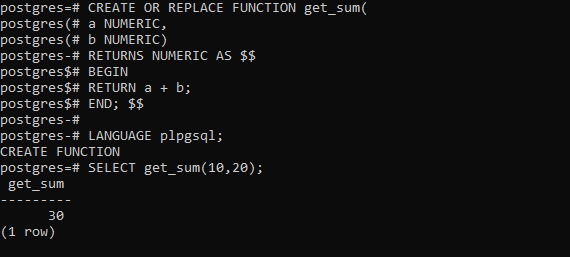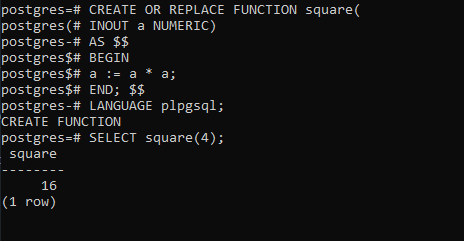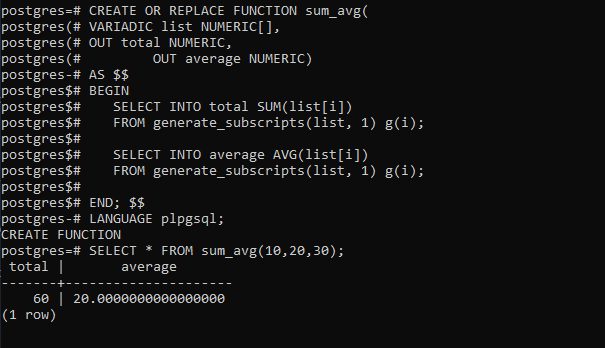PostgreSQL – Function Parameters

• Last Updated : 28 Aug, 2020

PostgreSQL has 4 types of function parameters as listed below:

• IN
• OUT
• INOUT

IN Parameter:

To better understand these function parameters let’s first define a function on which we will experiment with the above-mentioned function parameters as below:

CREATE OR REPLACE FUNCTION get_sum(
a NUMERIC,
b NUMERIC)
RETURNS NUMERIC AS \$\$
BEGIN
RETURN a + b;
END; \$\$

LANGUAGE plpgsql;

The get_sum() function accepts two parameters: a, and b,  and returns a numeric.

The data types of the two parameters are NUMERIC. By default, the parameter’s type of any parameter in PostgreSQL is IN parameter. You can pass the IN parameters to the function but you cannot get them back as a part of the result.

Example:

SELECT get_sum(10, 20);

Output:Out Parameter:

The OUT parameters are defined as part of the function arguments list and are returned back as a part of the result. For better understanding let’s define a function as below:

CREATE OR REPLACE FUNCTION hi_lo(
a NUMERIC,
b NUMERIC,
c NUMERIC,
OUT hi NUMERIC,
OUT lo NUMERIC)
AS \$\$
BEGIN
hi := GREATEST(a, b, c);
lo := LEAST(a, b, c);
END; \$\$

LANGUAGE plpgsql;

The hi_lo function accepts 5 parameters:

• Three IN parameters: a, b, c.
• Two OUT parameters: hi (high) and lo (low).

Inside the function, we get the greatest and least numbers of three IN parameters using GREATEST and LEAST built-in functions. Because we use the OUT parameters, we don’t need to have a RETURN statement. The OUT parameters are useful in a function that needs to return multiple values without the need of defining a custom type.

Example :

SELECT hi_lo(10, 20, 30);

Output:INOUT Parameter:

The INOUT parameter is the combination IN and OUT parameters. It means that the caller can pass a value to the function. The function then changes the argument and passes the value back as a part of the result.

The following square function accepts a number and returns the square of that number.

CREATE OR REPLACE FUNCTION square(
INOUT a NUMERIC)
AS \$\$
BEGIN
a := a * a;
END; \$\$
LANGUAGE plpgsql;

Example:

SELECT square(4);

Output:A function can accept a variable number of arguments with one condition that all the arguments have the same data type. The arguments are passed to the function as an array.

Example:

CREATE OR REPLACE FUNCTION sum_avg(
OUT total NUMERIC,
OUT average NUMERIC)
AS \$\$
BEGIN
SELECT INTO total SUM(list[i])
FROM generate_subscripts(list, 1) g(i);

SELECT INTO average AVG(list[i])
FROM generate_subscripts(list, 1) g(i);

END; \$\$
LANGUAGE plpgsql;

The sum_avg() function accepts a list of numbers, calculates the total and average, and returns both values.

SELECT * FROM sum_avg(10, 20, 30);

Output:My Personal Notes arrow_drop_up